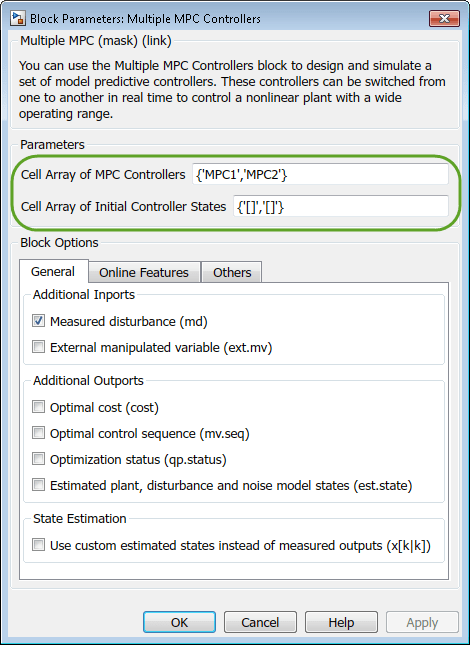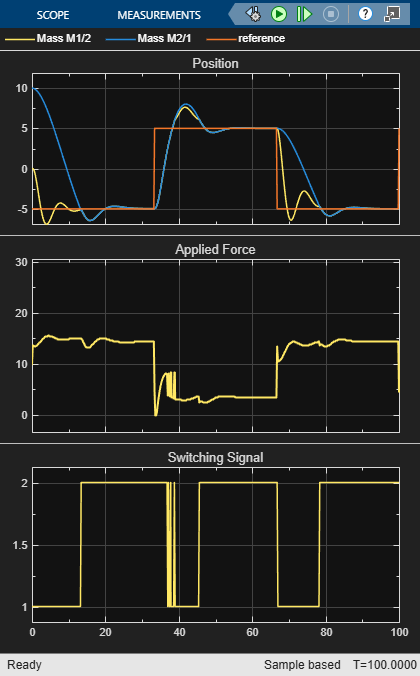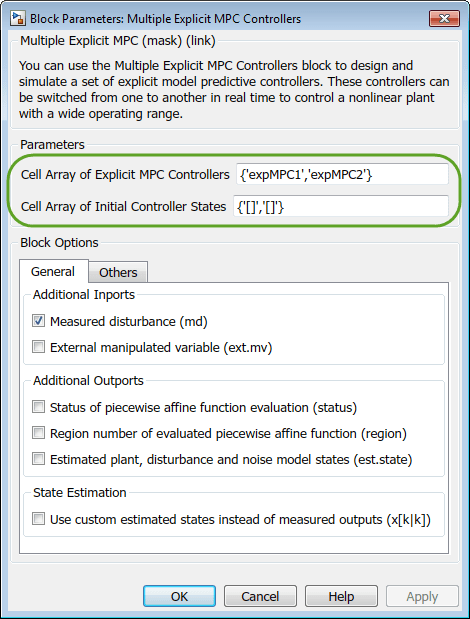Documentation

This is machine translation

Mouseover text to see original. Click the button below to return to the English version of the page.

Schedule Controllers at Multiple Operating Points

If your plant is nonlinear, a controller designed to operate in a particular target region may perform poorly in other regions. A common way to compensate is to create multiple controllers, each designed for a particular combination of operating conditions. You can then switch between the controllers in real time as conditions change. For more information, see Gain-Scheduled MPC.

The following example shows how to coordinate multiple model predictive controllers for this purpose.

Plant Model

The plant contains two masses, `M1` and `M2`, connected to two springs. A spring with spring constant `k1` pulls mass `M1` to the right, and a spring with spring constant `k2` pulls mass `M2` to the left. The manipulated variable is a force pulling mass `M1` to the left, shown as a red arrow in the following figure.Both masses move freely until they collide. The collision is inelastic, and the masses stick together until a change in the applied force separates them. Therefore, there are two operating conditions for the system with different dynamics.

The control objective is to make the position of `M1` track a reference signal, shown as a blue triangle in the previous image. Only the position of `M1` and a contact sensor are available for feedback.

Define the model parameters.

```M1 = 1; % masses M2 = 5; k1 = 1; % spring constants k2 = 0.1; b1 = 0.3; % friction coefficients b2 = 0.8; yeq1 = 10; % wall mount positions yeq2 = -10; ```

Create a state-space model for when the masses are not in contact; that is when mass `M1` is moving freely.

```A1 = [0 1; -k1/M1 -b1/M1]; B1 = [0 0; -1/M1 k1*yeq1/M1]; C1 = [1 0]; D1 = [0 0]; sys1 = ss(A1,B1,C1,D1); sys1 = setmpcsignals(sys1,'MV',1,'MD',2); ```

Create a state-space model for when the masses are connected.

```A2 = [0 1; -(k1+k2)/(M1+M2) -(b1+b2)/(M1+M2)]; B2 = [0 0; -1/(M1+M2) (k1*yeq1+k2*yeq2)/(M1+M2)]; C2 = [1 0]; D2 = [0 0]; sys2 = ss(A2,B2,C2,D2); sys2 = setmpcsignals(sys2,'MV',1,'MD',2); ```

For both models, the:

• States are the position and velocity of `M1`.

• Inputs are the applied force, which is the manipulated variable (MV), and a spring constant calibration signal, which is a measured disturbance (MD).

• Output is the position of `M1`.

Design MPC Controllers

Design one MPC controller for each of the plant models. Both controllers are identical except for their internal prediction models.

Define the same sample time, `Ts`, prediction horizon, `p`, and control horizon, `m`, for both controllers.

```Ts = 0.2; p = 20; m = 1; ```

Create default MPC controllers for each plant model.

```MPC1 = mpc(sys1,Ts,p,m); MPC2 = mpc(sys2,Ts,p,m); ```
```-->The "Weights.ManipulatedVariables" property of "mpc" object is empty. Assuming default 0.00000. -->The "Weights.ManipulatedVariablesRate" property of "mpc" object is empty. Assuming default 0.10000. -->The "Weights.OutputVariables" property of "mpc" object is empty. Assuming default 1.00000. -->The "Weights.ManipulatedVariables" property of "mpc" object is empty. Assuming default 0.00000. -->The "Weights.ManipulatedVariablesRate" property of "mpc" object is empty. Assuming default 0.10000. -->The "Weights.OutputVariables" property of "mpc" object is empty. Assuming default 1.00000. ```

Define constraints for the manipulated variable. Since the applied force cannot change direction, set the lower bound to zero. Also, set a maximum rate of change for the input force. These constraints are the same for both controllers.

```MPC1.MV = struct('Min',0,'Max',30,'RateMin',-10,'RateMax',10); MPC2.MV = MPC1.MV; ```

Simulate Gain-Scheduled Controllers

Simulate the performance of the controllers using the MPC Controller block.

```mdl = 'mpc_switching'; open_system(mdl) ```In the model, the Mass M1 subsystem simulates the motion of mass `M1`, both when moving freely and when connected to `M2`. The Mass M2 subsystem simulates the motion of mass M2 when it is moving freely. The mode selection and velocity reset subsystems coordinate the collision and separation of the masses.

The model contains switching logic that detects when the positions of `M1` and `M2` are the same. The resulting switching signal connects to the `switch` inport of the Multiple MPC Controllers block, and controls which MPC controller is active.

Specify the initial position for each mass.

```y1initial = 0; y2initial = 10; ```

To specify the gain-scheduled controllers, double-click the Multiple MPC Controllers block. In the Block Parameters dialog box, specify the controllers as a cell array of controller names. Set the initial states for each controller to their respective nominal value by specifying the states as `{'[],'[]'}`.Click OK.

Run the simulation.

```sim(mdl) ```
```-->Converting model to discrete time. -->Assuming output disturbance added to measured output channel #1 is integrated white noise. -->The "Model.Noise" property of the "mpc" object is empty. Assuming white noise on each measured output channel. -->Converting model to discrete time. -->Assuming output disturbance added to measured output channel #1 is integrated white noise. -->The "Model.Noise" property of the "mpc" object is empty. Assuming white noise on each measured output channel. ```To view the simulation results, open the signals scope.

```open_system([mdl '/signals']) ```Initially, `MPC1` moves mass `M1` to the reference setpoint. At about 13 seconds, `M2` collides with `M1`. The switching signal changes from 1 to 2, which switches control to `MPC2`.

The collision moves `M1` away from its setpoint and `MPC2` quickly returns the combined masses to the reference point.

During the subsequent reference signal transitions, when the masses separate and collide the Multiple MPC Controllers block switches between `MPC1` and `MPC2` accordingly. As a result, the combined masses settle rapidly to the reference points.

Compare with Single MPC Controller

To demonstrate the benefit of using two MPC controllers for this application, simulate the system using just `MPC2`.

Change `MPC1` to match `MPC2`.

```MPC1save = MPC1; MPC1 = MPC2; ```

Run the simulation.

```sim(mdl) ```When the masses are not connected, `MPC2` applies excessive force since it expects a larger mass. This aggressive control action produces oscillatory behavior. Once the masses connect, the control performance improves, since the controller is designed for this condition.

Alternatively, changing `MPC2` to match `MPC1` results in sluggish control actions and long settling times when the masses are connected.

Set `MPC1` back to its original configuration.

```MPC1 = MPC1save; ```

Create Explicit MPC Controllers

To reduce online computational effort, you can create an explicit MPC controller for each operating condition, and implement gain-scheduled explicit MPC control using the Multiple Explicit MPC Controllers block. For more information on explicit MPC controllers, see Explicit MPC.

To create an explicit MPC controller, first define the operating ranges for the controller states, input signals, and reference signals.

Create an explicit MPC range object using the corresponding traditional controller, `MPC1`.

```range = generateExplicitRange(MPC1); ```

Specify the ranges for the controller states. Both `MPC1` and `MPC2` contain states for:

• The position and velocity of mass `M1`.

• An integrator from the default output disturbance model.

When possible, use your knowledge of the plant to define the state ranges. For example, the first state corresponds to the position of `M1`, which has a range between -10 and 10.

Setting the range of a state variable can be difficult when the state does not correspond to a physical parameter, such as for the output disturbance model state. In that case, collect range information using simulations with typical reference and disturbance signals. For this system, you can activate the optional `est.state` outport of the Multiple MPC Controllers block, and view the estimated states using a scope. When simulating the controller responses, use a reference signal that covers the expected operating range.Define the state ranges for the explicit MPC controllers based on the ranges of the estimated states.

```range.State.Min(:) = [-10;-8;-3]; range.State.Max(:) = [10;8;3]; ```

Define the range for the reference signal. Select a reference range that is smaller than the `M1` position range.

```range.Reference.Min = -8; range.Reference.Max = 8; ```

Specify the manipulated variable range using the defined MV constraints.

```range.ManipulatedVariable.Min = 0; range.ManipulatedVariable.Max = 30; ```

Define the range for the measured disturbance signal. Since the measured disturbance is constant, specify a small range around the constant value, `1`.

```range.MeasuredDisturbance.Min = 0.9; range.MeasuredDisturbance.Max = 1.1; ```

Create an explicit MPC controller that corresponds to `MPC1` using the specified range object.

```expMPC1 = generateExplicitMPC(MPC1,range); ```
``` Regions found / unexplored: 4/ 0 ```

Create an explicit MPC controller that corresponds to `MPC2`. Since `MPC1` and `MPC2` operate over the same state and input ranges, and have the same constraints, you can use the same range object.

```expMPC2 = generateExplicitMPC(MPC2,range); ```
``` Regions found / unexplored: 5/ 0 ```

In general, the explicit MPC ranges of different controllers may not match. For example, the controllers may have different constraints or state ranges. In such cases, create a separate explicit MPC range object for each controller.

Validate Explicit MPC Controllers

It is good practice to validate the performance of each explicit MPC controller before implementing gain-scheduled explicit MPC. For example, to compare the performance of `MPC1` and `expMPC1`, simulate the closed-loop response of each controller using `sim`.

```r = [zeros(30,1); 5*ones(160,1); -5*ones(160,1)]; [Yimp,Timp,Uimp] = sim(MPC1,350,r,1); [Yexp,Texp,Uexp] = sim(expMPC1,350,r,1); ```
```-->Converting model to discrete time. -->Assuming output disturbance added to measured output channel #1 is integrated white noise. -->The "Model.Noise" property of the "mpc" object is empty. Assuming white noise on each measured output channel. ```

Compare the plant output and manipulated variable sequences for the two controllers.

```figure subplot(2,1,1) plot(Timp,Yimp,'b-',Texp,Yexp,'r--') grid on xlabel('Time (s)') ylabel('Output') title('Explicit MPC Validation') legend('Implicit MPC','Explicit MPC') subplot(2,1,2) plot(Timp,Uimp,'b-',Texp,Uexp,'r--') grid on ylabel('MV') xlabel('Time (s)') ```The closed-loop responses and manipulated variable sequences of the implicit and explicit controllers match. Similarly, you can validate the performance of `expMPC2` against that of `MPC2`.

If the responses of the implicit and explicit controllers do not match, adjust the explicit MPC ranges, and create a new explicit MPC controller.

Simulate Gain-Scheduled Explicit MPC

To implement gain-scheduled explicit MPC control, replace the Multiple MPC Controllers block with the Multiple Explicit MPC Controllers block.

```expModel = 'mpc_switching_explicit'; open_system(expModel) ```To specify the explicit MPC controllers, double-click the Multiple Explicit MPC Controllers block. In the Block Parameters dialog box, specify the controllers as a cell array of controller names. Set the initial states for each controller to their respective nominal value by specifying the states as `{'[],'[]'}`.Click OK.

If you previously validated the your explicit MPC controllers, then substituting and configuring the Multiple Explicit MPC Controllers block should produce the same results as the Multiple MPC Controllers block.

Run the simulation.

```sim(expModel) ```To view the simulation results, open the signals scope.

```open_system([expModel '/signals']) ```The gain-scheduled explicit MPC controllers provide the same performance as the gain-scheduled implicit MPC controllers.

```bdclose('all') ```